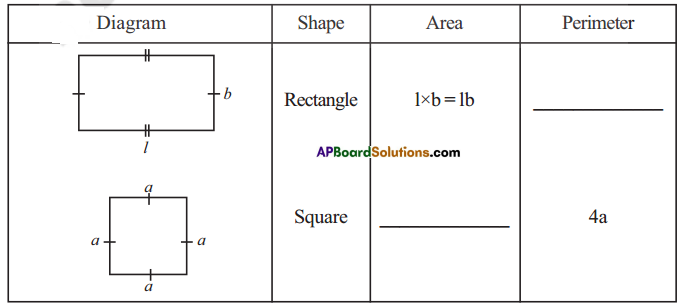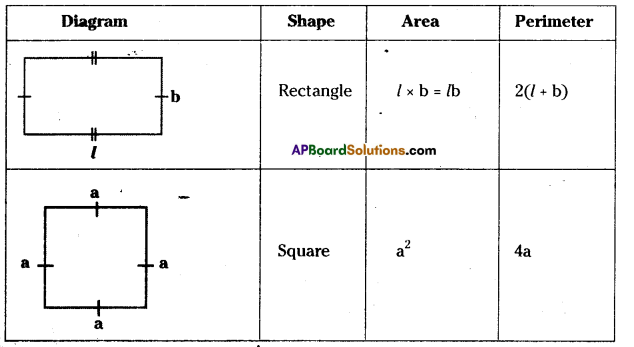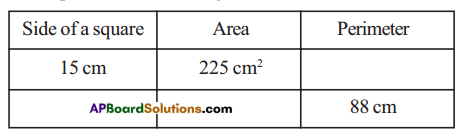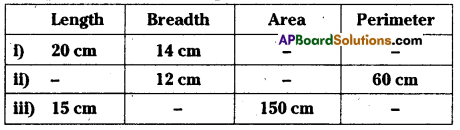AP State Syllabus AP Board 7th Class Maths Solutions Chapter 13 Area and Perimeter Ex 1 Textbook Questions and Answers.

## AP State Syllabus 7th Class Maths Solutions 12th Lesson Area and Perimeter Exercise 1Question 1.Solution:Question 2.
The measurements of some squares are given in the table below. However, they are incomplete. Find the missing information.Solution:
i) Perimeter = 4a = 4(15) = 60cm
ii) Perimeter = 4a = 88
a = $$\frac{88}{4}$$= 22
Side = 22cm
Area = a2 = (22)2 = 484 cm2Question 3.
The measurements of some rectangles are given in the table below. However, they are incomplete. Find the missing information.Solution:
Given:
i) Length = 20 cm
Area = l x b = 20 x 14 =280 cm2
Perimeter = 2(l + b) = 2(20 + 14)
= 2(34) = 68cm

ii) Given breadth = 12cm, perimeter = 60cm
Perimeter 2(l + b) 60 cm
= 2(l + 12) =60cm
2l + 24 =60cm
2l = 60 – 24 = 36
2l = $$\frac{36}{2}$$ = 18cm
Area = l x b = 18 x 12 = 216cm2iii) Given length = 15cm, Area = 150 cm
Area = l x b = 15 x b = 150
⇒ b = $$\frac{150}{15}$$ = 10cm
Perimeter = 2(l + b) = 2(15 + 10)
= 2(25) =50cm